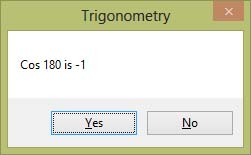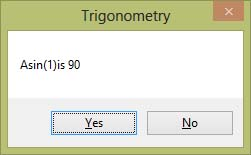# Visual Basic 2017 Lesson 19: Trigonometric Functions

#### [Lesson 18]<<[Contents]>>[Lesson 20]

In the previous lesson, we have learned how to write code using various mathematical functions in Visual Basic 2017. In this lesson, we shall proceed to learn how to work with a group of mathematical functions that deal with angles and lengths of a polygon, the trigonometric functions. The three basic trigonometric functions are Sin, Cos, and Tan which stand for sine, cosine, and tangent. We also deal with the inverse trigonometric functions Asin, Acos, and Atan respectively.

### 19.1 The Sin function

The Sin function returns the sine value of an angle. We need to convert the angle to radian as Visual Basic 2017 cannot deal with an angle in degree. The conversion is based on the following equation:

π radian= 180º

so 1º=π/180 radian

The issue is how to get the exact value of π? We can use π=3.14159 but it will not be accurate. To get the exact value of π, we use the arc sine function, i.e. is Asin.

Using the equation   sin(π/2)=1, so Asin(1)=π/2, therefore, π=2Asin(1)

The syntax of  the Sin function in Visual Basic 2017 is

Math.Sin(Angle in radian)

#### Example 19.1

In this example, we use pi to represent π and assign the value of π using the formula pi = 2*Math.Asin(1). We use the function Round to round the value of sine to four decimal places.

```Private Sub Button1_Click(sender As Object, e As EventArgs) Handles Button1.Click
Dim pi As Single
pi = 2*Math.Asin(1)
MsgBox("Sin 90 is" & Math.Round(Math.Sin(pi / 2), 4))
End Sub
```

The Output

### 19.2 The Cos function

In Visual Basic 2017, the Cos function returns the Cosine value of an angle. The syntax is
Math.Cos(Angle in radian)

#### Example 19.2

```Private Sub Button1_Click(sender As Object, e As EventArgs) Handles Button1.Click
Dim pi As Single
pi = 2*Math.Asin(1)
MsgBox("Cos 180 is " & Math.Round(Math.Cos(pi), 4))
End Sub
```

The OutputFigure 19.2

### 19.3 The Tan function

In Visual Basic 2017, the Tan function returns the tangent value of an angle. The syntax is

Math.Tan(Angle in radian)

#### Example 19.3

```Private Sub Button1_Click(sender As Object, e As EventArgs) Handles Button1.Click
Dim pi As Single
pi = 2 * Math.Asin(1)
MsgBox("Tan 45 is " & Math.Round(Math.Tan(pi / 4)), 4)
End Sub```

The Output### 19.4 The Asin Function

The Asin function returns the value of arcsine (inverse sine ) and it represents the angle that corresponds to the sine value. For example, sin90º = 1,  so asin(1)=90º

In Visual Basic 2017, the value of arcsine is expressed in terms of radian. To convert the value to degree, we use the formula 1 radian=180º/π, where π=2Asin(1).

#### Example 19.4

```Private Sub Button1_Click(sender As Object, e As EventArgs) Handles Button7.Click
Dim pi As Single
pi = 2 * Math.Asin(1)
MsgBox("Asin(1)is " & Math.Round(Math.Asin(1) * 180 / pi), 4)
End Sub
```

The OutputFigure 19.4

### 19.5 The Acos Function

In Visual Basic 2017, the Acos function returns the value of arc cosine (inverse cosine ) and it represents the angle that corresponds to the cos value. For example, cos90º = 0,  so acos(0)=90º

#### Example 19.5

```Private Sub Button1_Click(sender As Object, e As EventArgs) Handles Button7.Click
Dim pi As Single
pi = 2 * Math.Asin(1)
MsgBox("Asin(1)is " & Math.Round(Math.Acos(1) * 180 / pi), 4)
End Sub
```Figure 19.5

### 19.6 The Atan Function

In Visual Basic 2017, the Atan function returns the value of arc tangent (inverse tangent) and it represents the angle that corresponds to the tan value. For example, tan45º = 1,  so atan(1)=45º

#### Example 19.6

```Private Sub Button1_Click(sender As Object, e As EventArgs) Handles Button7.Click
Dim pi As Single
pi = 2 * Math.Asin(1)
MsgBox("Asin(1)is " & Math.Round(Math.Atan(1) * 180 / pi), 4)
End Sub
```

The OutputFigure 19.6

### 19.7 Applications

Trigonometric functions can be used to solve various mathematical problems. Common examples are problems involving the sine rule, the cosine rule, trigonometric identities, trigonometric equations, the projectile equation and more.

#### Example 19.7 The Sine Rule

For example, in a triangle ABC, give angle  A=60 and angle B=30 respectively, length BC denoted by a=4  you can calculate length AC (denoted by b).

The formula is

a/sinA=b/sinB, so b=asinB/sinA

```Private Sub Button8_Click(sender As Object, e As EventArgs) Handles Button8.Click
Dim pi As Single
Dim A As Single, B As Single, x As Single
pi = 2 * Math.Asin(1)
A = 60 * pi / 180
B = 40 * pi / 180
x = 4
MsgBox("AC= " & Math.Round((x * Math.Sin(A) / Math.Sin(B)), 4))
End Sub
```

The Output